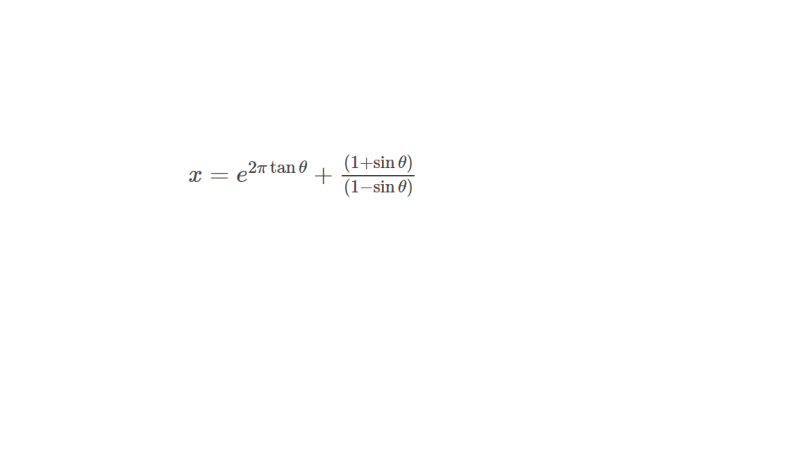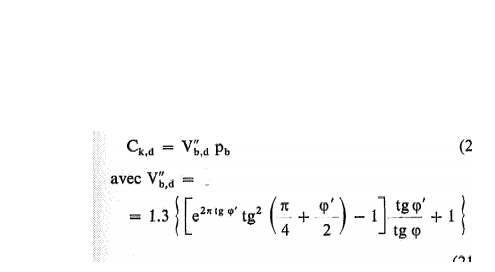×
INTELLIGENT WORK FORUMS
FOR ENGINEERING PROFESSIONALS

Are you an
Engineering professional?
Join Eng-Tips Forums!
• Talk With Other Members
• Be Notified Of Responses
• Keyword Search
Favorite Forums
• Automated Signatures
• Best Of All, It's Free!

*Eng-Tips's functionality depends on members receiving e-mail. By joining you are opting in to receive e-mail.

#### Posting Guidelines

Promoting, selling, recruiting, coursework and thesis posting is forbidden.

# Hi, I have an equation which is sh

## Hi, I have an equation which is sh

(OP)
Hi,
I have an equation which is shown below, which says qc (cone resistance) = .....
Now i need to calculate the effective angle of internal friction from the below formula. So i need to make a new formula
which starts with φ'= ......
qc= 1.3 × exp[2π tanφ'] × tan2[(π/4)+ fracφ'2] × σv0
And i have no idea what is this fracφ'2.
I am stuck with this value.
I cannot use any other formula to calculate φ'. It is that i have to use this formula./
Does anyone know how to reverse the equation so that i can calculate effective angle of internal friction?
The qc value and effective stress are given.

### RE: Hi, I have an equation which is sh

From where does this equation of yours come? Source?

### RE: Hi, I have an equation which is sh

(OP)
This is the equation for calculating φ' (effective friction angle) by "De Beer" method. In Belgium practice, they follow this method for calculating the pile bearing capacity. And i am in the starting stage of the calculation. I cannot go any further if i dont have an equation for φ'. I have to follow this method, because the project is in Belgium.
I have tried to simplify this equation and now i am getting likeLet θ be φ' in the above equation and x is a constant.
Now i need to solve for θ in terms of x.
Does anyone know how to do it?

### RE: Hi, I have an equation which is sh

Unless you luck out with Wolfram Alpha or some other symbolic solver, I would use plug into Excel or MAthcad and numerically solve for it.

It seems odd that an equation in general use for this application wouldn't already have a symbolic solution or approximation.

TTFN (ta ta for now)
I can do absolutely anything. I'm an expert! https://www.youtube.com/watch?v=BKorP55Aqvg
FAQ731-376: Eng-Tips.com Forum Policies forum1529: Translation Assistance for Engineers Entire Forum list http://www.eng-tips.com/forumlist.cfm

### RE: Hi, I have an equation which is sh

This is one of the means to estimate the friction angle Ø using cone bearing.
qc= 1.3 × exp[2π tanØ] × tan2[(45 + Ø/2] × σv0)
σv0 = ɣ'z (overburden pressure) and q'c are both "effective" stresses and you must know them..

You can simplfy the equation (σv0)/(1.3*σv0)= exp[2π tanØ] × tan2[(45 + Ø/2]

Now, the solve the implicit function with numerical analysis.. One of the methods, trial ,..

In your final formula, take natural log of both sides ln x = 2π tanØ + 2ln ( π/4+ Ø/2)

tanØ = (ln x-2ln ( π/4+ Ø/2))/( 2π ) now start with trial Ø=30 degr but in radians (π /6) you will get another Ø
and try with new Ø.. The formula will converge after 4-5 trials..

Or you may look to the Figure 3-22 in the famous book Foundation Analysis and Design Joseph E. Bowles page 180.

I have attached the graph..

### RE: Hi, I have an equation which is sh

(OP)
Thank you so much for your help.
But in the above comment, when we take log on both sides, tan2 is missing from your equation.

And also in the attached graph, according to De Beer, the equation is used on the condition that : when Ø´ is greater than Ø(provided). Inorder to find out this Ø´ i have use the below equation.And for calculating the effective friction angle, i need to convert the above formula into Ø´= ....
Where the values of Ø , Ckd(=qc) and σv0 will be provided.

### RE: Hi, I have an equation which is sh

Typing mistakes...yes tan**2 is forgotten...

qc= 1.3 × exp[2π tanØ] × tan2[(45 + Ø/2] × σv0)

qc/(1.3σv0 ) = exp[2π tanØ] × tan2[(45 + Ø/2]

C= exp[2π tanØ] × tan2[(45 + Ø/2]

Now take the natural log of both sides,

ln C= 2π tanØ + 2*ln tan [(45 + Ø/2] another way, define function Ω = 2π tanØ + 2*ln tan [(45 + Ø/2] -Ln C

The value of Ø which the function Ω = 0 will be the answer..

Regarding your quote: Vb" = Ckd /Pb where Pb = y'z (overburden pressure) and Ckd (cone resistance) are both "effective" stresses ..

### RE: Hi, I have an equation which is sh

(OP)
Thank you so much for your help.

But i am sorry beacuse still i couldnt get an equation for Ø
When Ω = 0 ,
0= 2π tanØ + 2tan [(45 + Ø/2] - LnC
In this above equation , Suppose C= 20, How to get a value for Ø
I need to get the Ø value in terms of C. There are still two Ø terms in this equation.
I cannot go for derivation again, because if derivate again, this C will become zero.
I need to calculate the values of Ø for different values of C.
Thank you so much.

### RE: Hi, I have an equation which is sh

C is a constant used for simplification of the formula...In this case , C=qc/(1.3σv0 )..You must know the σv0 (overburden pressure) and qc (cone resistance) both effective stress...

0= 2π tanØ + 2tan [(45 + Ø/2] - LnC

This nonlinear eq. can be solved with iterative methods. For example, Bisection method,

Give arbitrary value to Ø .. Consider two points, (a, f (a)), and (b, f (b)) which the function having (+ and - ) opposite signs .Thus, we know that there is a root of f in the interval (a, b). Let c be the midpoint of the interval [a, b], so that c = (a+b)/2, and evaluate f (c). One of the following is true: Either f (c) = 0, f (a) and
f (c) have opposite signs, or f (c) and f (b) have opposite signs. Now try d=(a+c)/2 or d=(b+c)/2 ..a or b will be decided so that they must opposite sign with c...After a few iteration , the diffrence will be negligible and you will find Ø .

### RE: Hi, I have an equation which is sh

(OP)
Thank you so much for your help.
I will try this method

#### Red Flag This Post

Please let us know here why this post is inappropriate. Reasons such as off-topic, duplicates, flames, illegal, vulgar, or students posting their homework.

#### Red Flag Submitted

Thank you for helping keep Eng-Tips Forums free from inappropriate posts.
The Eng-Tips staff will check this out and take appropriate action.

#### Resources

White Paper - How ESI is Helping Move New Medical Device Product to Market Quicker & More Cost Effic
Early Supplier Involvement has long been a strategy employed by manufacturers to produce innovative products. Now, it almost seems like a necessity. Because decisions made in the design phase can positively affect product quality and costs, this can help add value to OEM bottom lines. This white paper will discuss many facets of ESI, including why itâ€™s so valuable today, what challenges limit the benefits of ESI, how cost is impacted, and more. Download Now
White Paper - Moving to a Driverless Future
This white paper describes what we see as the best practices to support a sustainable engineering process for autonomous vehicle design. It exposes how to use simulation and testing in common frameworks to enable design exploration, verification and validation for the development of autonomous cars at a system, software and full-vehicle level to drive a mature product development process for automated driving. Download Now
Guide - When to Outsource Manufacturing to a Service Provider
As a 3D printer owner, you may be already reaping the benefits of 3D printing, such as rapid production, design freedoms and easy access to fresh prints of the machine. We dive into scenarios to help you decide when you need to outsource your project to a service provider. Download Now
Research Report - How Engineers are Using Remote Access
Remote access enables engineers to work from anywhere provided they have an internet connection. We surveyed our audience of engineers, designers and product managers to learn how they use remote access within their organizations. We wanted to know which industries have adopted remote access, which software they are using, and what features matter most. Download Now

Close Box

# Join Eng-Tips® Today!

Join your peers on the Internet's largest technical engineering professional community.
It's easy to join and it's free.

Here's Why Members Love Eng-Tips Forums:

•Talk To Other Members
• Notification Of Responses To Questions
• Favorite Forums One Click Access
• Keyword Search Of All Posts, And More...

Register now while it's still free!